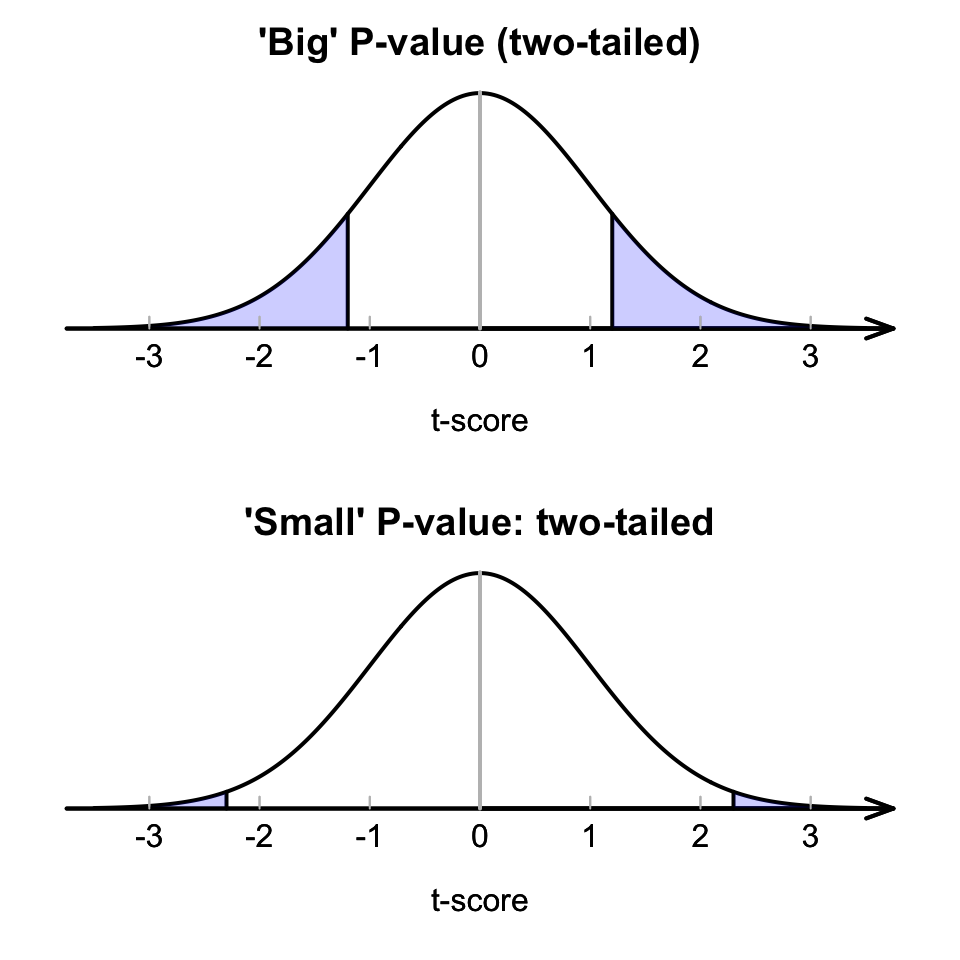## 27.6 Making decisions with $$P$$-values

$$P$$-values tells us the likelihood of observing the sample statistic (or something more extreme), based on the assumption about the population parameter being true. In this context, the $$P$$-value tells us the likelihood of observing the value of $$\bar{x}$$ (or something more extreme), just through sampling variation (chance) if $$\mu=37$$. The $$P$$-value is a probability, albeit a probability of something quite specific, so it is a value between 0 and 1. Then:

• ‘Big’ $$P$$-values mean that the sample statistic (i.e., $$\bar{x}$$) could reasonably have occurred through sampling variation, if the assumption about the parameter (stated in $$H_0$$) was true (Fig. 27.7, top panel): The data do not contradict the assumption ($$H_0$$).

• ‘Small’ $$P$$-values mean that the sample statistic (i.e., $$\bar{x}$$) is unlikely to have occurred through sampling variation, if the assumption about the parameter (stated in $$H_0$$) was true: (Fig. 27.7, bottom panel): The data contradict the assumption.

What is meant by ‘small’ and ‘big?’ It is arbitrary: no definitive rules exist. Commonly, a $$P$$-value smaller than 1% (that is, smaller than 0.01) is usually considered ‘small,’ and a $$P$$-value larger than 10% (that is, larger than 0.10) is usually considered ‘big.’ Between the values of 1% and 10% is often a ‘grey area.’FIGURE 27.7: A picture of large (top) and small (bottom) $$P$$-value situations

Traditionally, a $$P$$-value is ‘small’ if it is less than 5% (less than 0.05), and ‘big’ if greater than 5% (greater than 0.05). However, again this is arbitrary, and binary decision making (either big or small) is unreasonable. More reasonably, $$P$$-values should be interpreted as providing varying strength of evidence in support of the alternative hypothesis $$H_1$$ (Table 28.1). These are not definitive, but are only guidelines. Of course, conclusions should be written in the context of the problem.

For one-tailed tests, the $$P$$-value is half the value of the two-tailed $$P$$-value.

SPSS always produces two-tailed $$P$$-values, usually calls them ‘Significance values,’ and labels them as Sig., and sometimes explicitly notes that they are two-tailed.

For the body-temperature data then, where $$P<0.001$$, the $$P$$-value is very small, so there is very strong evidence that the population mean body temperature is not $$37.0^\circ\text{C}$$.